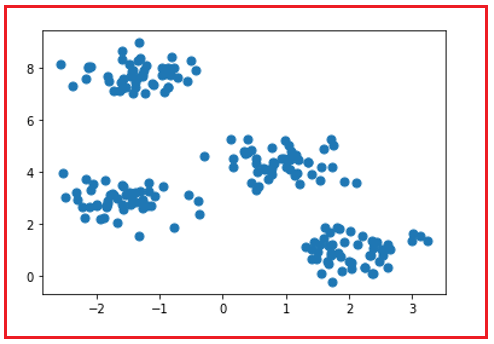# K-Means Clustering in Machine Learning

## K-Means Clustering in Machine Learning

In this article, I am going to discuss K-Means Clustering in Machine Learning with Examples. Please read our previous article where we discussed Predictive Models in Machine Learning with Examples.

##### K-Means Clustering in Machine Learning

Let’s pretend we’re starting a company to sell people services. Business is booming, and we’ve amassed a database of over ten thousand consumers in just a few months. We want to keep our clients while also increasing the amount of money we make each customer. As a result, we want to give a discount to the clients in our database. There are two possibilities:

1. Customers should all get the same deal.
2. Prepare discounts that are unique to each customer.

The first option is the simplest. A deal like “5% off all services” would suffice. It is, however, less efficient and profitable than customer-specific deals. Additionally, customer-specific promotions are more likely to appeal to customers. Some clients prefer a single-item discount, while others prefer a buy-one-get-one-free promotion.

Service X is purchased by some consumers on weekends, while others purchase it on Monday mornings. Depending on the size of the company and the number of customers, we can provide a long list of additional choices. We make the decision to prepare customer-specific offers. The next step is to figure out what kind of bargains you’ll be offering. We can’t just offer each buyer a different deal. That is inexcusably unmanageable.

Detecting and grouping customers with similar interests or purchase behaviour could be a good option. Customer preferences, tastes, hobbies, customer-service combinations, and other factors can be used to group customers. Assume that each client in your database contains the following information:

1. Age and location of the customer
2. Amount spent on average
3. Purchase quantity on average
5. Purchase date and kind

This list is easily expandable. Manually grouping customers is really challenging. Then we enlist the assistance of machine learning. Clustering is the process of putting like customers together.

Cluster analysis, often known as clustering, is the problem of arranging a set of objects so that objects in the same group (called a cluster) are more comparable (in some sense) to those in other groups (clusters).

Customers are the objects in our scenario. Clustering is unsupervised, which means samples are not labelled (data points). Many industries employ clustering for example –

1. Customer Segmentation
2. Image Segmentation
3. Detecting anomalies
4. Detection outliers

The most popular algorithm used for clustering is known as the K-means algorithm. Let’s understand the algorithm in a bit more detail now –

The goal of K-means clustering is to divide data into k clusters so that data points in the same cluster are similar and data points in other clusters are further apart.

The distance between two locations determines their similarity. The distance can be measured in a variety of ways. One of the most often used distance metrics is the Euclidean distance (Minkowski distance with p=2). The diagram below illustrates how to determine the Euclidean distance between two points in a two-dimensional space. The square of the difference between the x and y coordinates of the locations is used to calculate it.

Other distance measurements include cosine similarity, average distance, and so forth. The core of k-means clustering is the similarity measure. To choose the optimum measurement type, it is necessary to have solid domain expertise.

K-means clustering aims to reduce distances inside a cluster while increasing distances between clusters.

Let’s have a look at a step-by-step example of clustering implementation in practice –

1. Import Necessary Libraries –

```# Import necessary libraries
import numpy as np
import pandas as pd
import matplotlib.pyplot as plt
from sklearn.datasets import make_blobs
from sklearn.cluster import KMeans
```

2. Scikit-learn has a lot of tools for creating synthetic datasets, which are great for testing machine learning algorithms. I’m going to use the make blobs method.

```X, y = make_blobs(n_samples = 200, centers=4, cluster_std = 0.5, random_state = 0)
plt.scatter(X[:, 0], X[:, 1], s=50)
```3. The data is then fitted using a KMeans object.

```kmeans = KMeans(n_clusters = 4)
kmeans.fit(X)
```

4. The dataset can now be divided into clusters.

```y_pred = kmeans.predict(X)
plt.scatter(X[:, 0], X[:, 1], c = y_pred, s=50)
```

Real-world datasets are far more complex, with clusters that are not easily distinguished. The algorithm, on the other hand, functions in the same way.

The number of clusters cannot be determined using the K-means technique. When constructing the KMeans object, we must define it, which might be a difficult operation.

It is an iterative procedure to use K-means. The approach is based on the expectation-maximization method. It operates by doing the following stages after determining the number of clusters:

1. Choose centroids (cluster centres) at random for each cluster.
2. Calculate the distance between the centroids and all data points.
3. Assign data points to the cluster that is closest to them.
4. Take the mean of all data points in the cluster to find the new centroids of each cluster.
5. Steps 2, 3, and 4 should be repeated until all points converge and the cluster centres cease moving.

Note that the initial centroids are picked at random, which may result in somewhat different end clusters. The n init argument in scikit learn helps to solve this problem. The k-means method will run “n init” times with different beginning centroids, with n init consecutive runs determining the final results.

In the next article, I am going to discuss TF-IDF in Machine Learning with Examples. Here, in this article, I try to explain K-Means Clustering in Machine Learning with Examples. I hope you enjoy this K-Means Clustering in Machine Learning with Examples article.